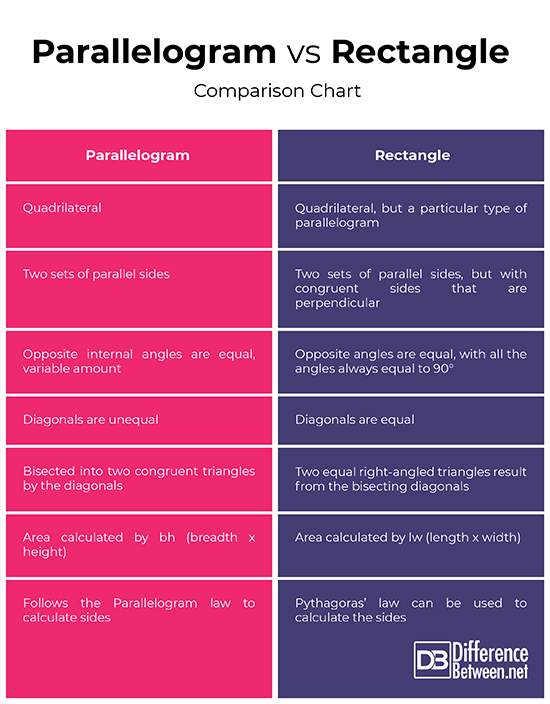#Difference Between Similar Terms and Objects

# Difference Between Parallelogram and Rectangle

Geometry addresses the classification of shapes and figures, which can also be described as the spatial orientation of an object. There is a wide array of different geometrical shapes, including the two-dimensional quadrilaterals. This refers to all four-sided geometrical shapes, which are further divided into four categories, namely trapezoids, isosceles trapezoids, kites, and parallelograms. These are all simple shapes that do not intersect itself and consists of an area enclosed by four sides.## What is a Parallelogram?

A parallelogram is classified as a closed quadrilateral figure with congruent or similar opposite sides that are parallel, also known as a quadrangle. The two parallel sides are known as the bases of a parallelogram, with the distance between the pair referred to as the height. The area of a parallelogram can be described as (1/2)h(2b), or rather bh, where h is height, and b denotes base. Another feature distinguishing parallelograms is the two pairs of parallel lines. The diagonals are another feature to consider; when drawn between opposite angles, the lines exactly bisect each other. Each of these diagonals tends to divide the parallelogram into two equal triangles, while both diagonals crossing divides it into four triangles, opposite triangles being equal. When the squares of the sides are added, it is the same as the sum of the diagonals. A parallelogram also has supplementary adjacent angles.## What is a rectangle?

A rectangle is often described as a special case of the parallelogram, since it has similar properties but with the height being the same as one of the parallel sides. This means the formula for a rectangle is lw (length x width) instead of bh. Rectangles also have two opposite parallel sides, although it also has perpendicular sequential sides, which means opposite angles are always 90°. The diagonals always bisect each other and result in line sections of equal length. In other words, a parallelogram that possesses equal opposite sides and 90° angles, is called a rectangle.

## Parallelogram Vs. Rectangle

### 1. Classification

These are both quadrilaterals, with a rectangle being classified as a type of parallelogram. Parallelograms and rectangles both have two sets of parallel sides, although a rectangle has consecutive sides that are perpendicular.

### 2. Angles

The opposite internal angles of both a parallelogram and rectangle are equivalent. The main difference is that a rectangle always has angles of 90°, while that of a parallelogram may vary.  In other words, the angles of a rectangle are always equal, or equiangular.

### 3. Diagonals

In the case of a parallelogram, the diagonals are unequal, and it bisects the shape into two congruent triangles. A rectangle has equal diagonals, which bisects the rectangle into two equal right triangles.

### 4. Formulas

The formula for calculating the area of parallelograms is bh (breadth x height), while the area of a rectangle is calculated by lw (length x width).

There is a ‘Parallelogram law’ that applies to parallelograms, where the sum of the squares of all the sides is equivalent to the sum of the squares of the diagonals. Rectangles, on the other hand, obey ‘Pythagoras’ law’, where the squares of the two adjacent sides added together is the same as the square of the diagonal.

### Parallelogram vs Rectangle : Comparison Chart## Summary of Parallelogram Vs. Rectangle

There are certain criteria that identify a quadrilateral shape as a parallelogram. The most obvious is the presence of two pairs of parallel sides. A rectangle is known as a special case of a parallelogram since it adheres to the basic classification of a parallelogram, but it has features that set it apart. This includes the opposite sides of equal length intersecting at 90° in all cases. The diagonals are thus equal, and divides the rectangle into right triangles, whereas the diagonals of a parallelogram are not equal and bisect it into two congruent triangles with angles depending on that of the parallelogram.

Latest posts by Mardi Stratuss (see all)

### Search DifferenceBetween.net :

Custom Search

Help us improve. Rate this post!Loading...Email This Post : If you like this article or our site. Please spread the word. Share it with your friends/family.

Please note: comment moderation is enabled and may delay your comment. There is no need to resubmit your comment.

## References :